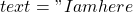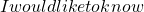Machine LearningNatural Language Processing

# N-Grams in Natural Language Processing## N-Grams

N-Grams is a Statistical Language Model that refers to a sequence of N words. A single word (natural) is a unigram, two words (natural language) is a bigram, three words (natural language processing) is a tri-gram and so on. These N-gram probabilities are useful to build applications based on probabilities. N-gram model is mainly used for text completion, speech recognition and auto-correct.

N-grams, however, follows Markov’s assumptions. According to this assumption, the probability of current word depends only on the previous N-words.

## N-Gram Model

For the purpose of understanding N-grams properly, let’s consider few sentences. We call these sentences our corpus, i.e., the data we train our model on.

<s> I am here </s>
<s> who am I </s>
<s> I would like to know </s>

Note: <s> refers to beginning of a sentence, whereas, </s> denotes the end of the sentence.

Using these sentences, we can find the bigram model for the corpus.

#### Bigram Model

The bigram model can be calculated by the following formula:where, P is the probability for word wi to occur after wi-1 and C is the count of the words. Based on this, we can find the bigram probabilities for any 2 words. For example:Similarly, we can find other probabilities and use them for our applications. Since our corpus is very small, we cannot make any meaningful sentence. However, we can try to find the probability of “I am I”, only for demonstrating the use of bigram model.Thus, the probability for sentence “I am I” is 1/27 based on our corpus.

## N-Grams using NLTK

NLTK provides with a simple tool to find all the N-gram pairs for a given sentence. Additionally, it allows to specify the value of N. A value of N = 2 provides bigrams, value of N = 3 provides trigrams and so on. Later, we can use a dictionary Counter to find the probabilities of these n-grams.

from nltk import ngrams

# Replaced <s> to # and </s> to# Who am I"
result = [ngram for ngram in ngrams(text.split() , n=2)]
print("Bigrams:", result)

from collections import Counter
print("Counter: ", dict(Counter(result)))

Output

Bigrams: [("#", "I"), ("I", "am"), ("am", "here"), ("here", "$"), ("$", "#"), ("#", "Who"), ("Who", "am"), ("am", "I"), ("I", "$"), ("$", "#"), ("#", "I"), ("I", "would"), ("would", "like"), ("like", "to"), ("to", "know"), ("know", "$")] Counter: {("#", "I"): 2, ("I", "am"): 1, ("am", "here"): 1, ("here", "$"): 1, ("$", "#"): 2, ("#", "Who"): 1, ("Who", "am"): 1, ("am", "I"): 1, ("I", "$"): 1, ("I", "would"): 1, ("would", "like"): 1, ("like", "to"): 1, ("to", "know"): 1, ("know", "\$"): 1} 

Thus, we can now leverage the concept of N-grams to build applications.

Further in the series: Part Of Speech Tagging – POS Tagging in NLP

## ReferencesDeep Mehta is a Machine Learning Engineer, Web Developer and Technical Blogger, currently pursuing Masters in Computer Science from New York University. In addition to being one of the founders of byteiota.com, he is an enthusiast in the domain of Artificial Intelligence. When he isn't working, he is either reading or writing a blog.